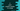# C program to print a random number in a range# Introduction :

In this tutorial, we will learn how to print a random number in a range in C. In our program, the user will enter the lowest and the highest number and it will print out five random numbers in this range.

C has one inbuilt function called rand() to find out a random number within a range from 0 to RANDMAX. The value of _RANDMAX_ may vary but it is at least 32767. This function is defined in stdlib.h file. So, we need to import this header file at the top of our program. Now, if we want to find out a random number in a range, we cannot find it directly by using the rand() function. We need to use a different formula as below :

``(rand() % (u – l + 1)) + l``

Here,

u is the upper limit. l is the lower limit.

So, if we want to find a random number between 0 to 9, it will be :

``(rand() % (9 - 0 + 1)) + 0``

or

``rand() % 10``

Similarly, we can find out a random number in a given range using the same formula.

## C program :

Now, let’s try to write down one program to print 5 random numbers in a range. The program will take the upper, lower limit from the user and using one loop, it will print out five random numbers.

``````#include <stdio.h>
#include <stdlib.h>

int main()
{
//1
int upperLimit;
int lowerLimit;
int i,n;

//2
printf("Enter the lower limit : \n");
scanf("%d", &lowerLimit);

printf("Enter the upper limit : \n");
scanf("%d", &upperLimit);

//3
printf("Five random numbers within %d and %d are :\n", lowerLimit, upperLimit);

for (i = 1; i < 6; i++)
{
n = (rand() % (upperLimit - lowerLimit + 1)) + lowerLimit;
printf("Number %d : %d\n",i,n);
}
return 0;
}``````

### Explanation :

_The commented numbers in the above program denote the steps number below :

_1. Create two integer variables upperLimit and lowerLimit to hold the upper and lower limit of the random numbers. Also, create two more variables to use in the for loop.

1. Ask the user to enter the lower limit. Read and store it in lowerLimit variable. Similarly, read and store the upper limit in the upperLimit variable.
2. Now, print out the random numbers. We are using one for loop here to print out five random numbers in the range. n is the random number calculated using the same formula defined above.

Few sample output of the above program is as below :

``````Enter the lower limit :
1
Enter the upper limit :
10
Five random numbers within 1 and 10 are :
Number 1 : 8
Number 2 : 10
Number 3 : 4
Number 4 : 9
Number 5 : 1

Enter the lower limit :
0
Enter the upper limit :
5
Five random numbers within 0 and 5 are :
Number 1 : 1
Number 2 : 1
Number 3 : 5
Number 4 : 2
Number 5 : 4

Enter the lower limit :
0
Enter the upper limit :
100
Five random numbers within 0 and 100 are :
Number 1 : 41
Number 2 : 65
Number 3 : 31
Number 4 : 41
Number 5 : 19``````

### Conclusion :

Try to run the above program to find random numbers with a different range. If you have any queries, please drop one comment below. Happy Coding :)